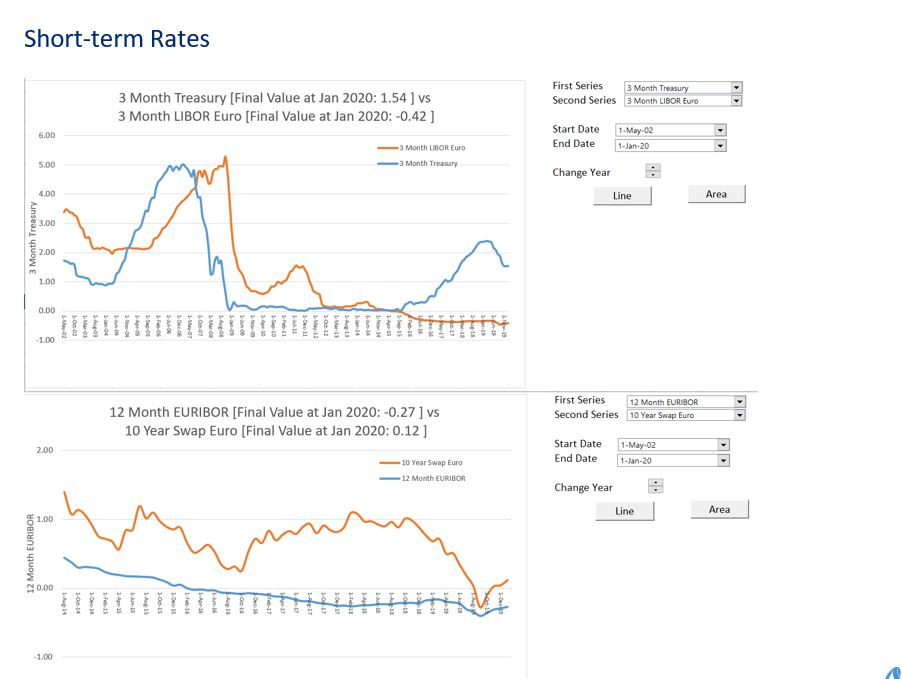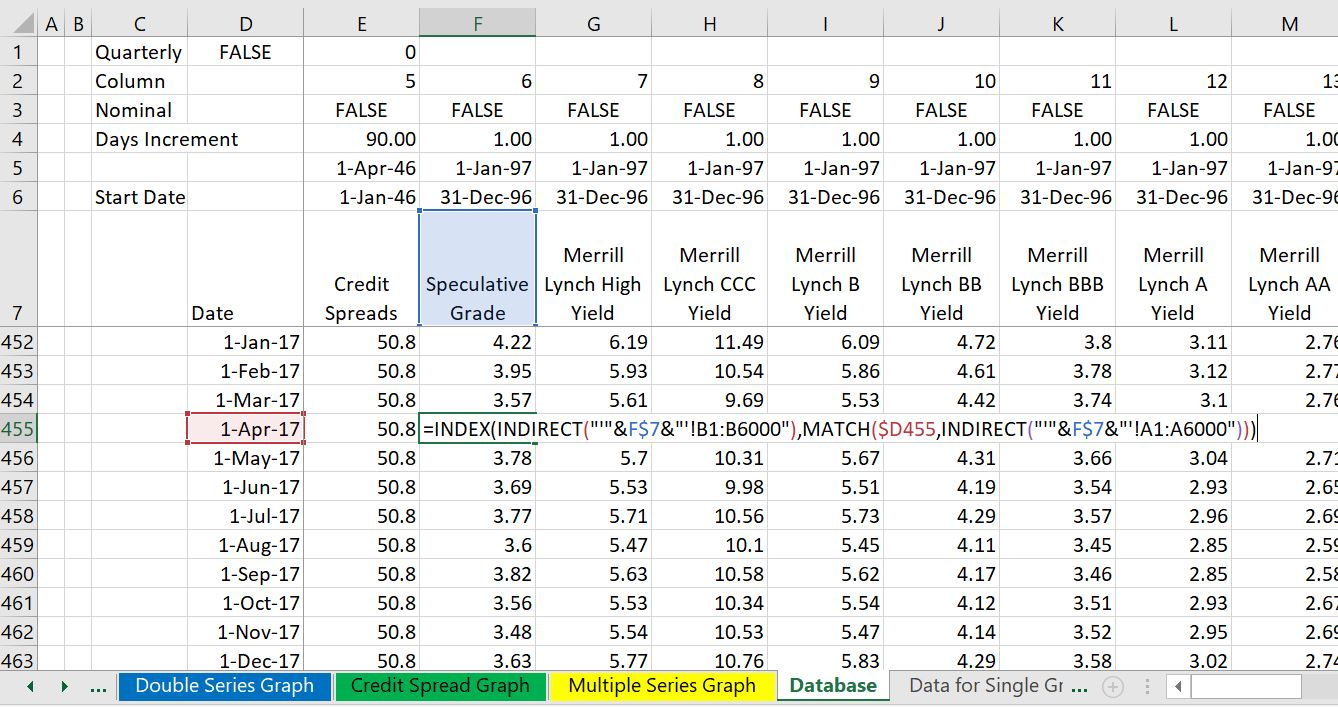# Interest Rate and Credit Spread Analysis from FRED

This page describes how you can quickly create an interest rate and credit spread database with automatic updates. The database retrieves information on interest rates and presents flexible graphs on credit spreads, the term structure of interest rates, interest rates in different currencies and other items.  You can mix and match the interest rate statistics to compare credit spreads with long-term or short-term interest rates. As with other database files, the process uses the WORKBOOKS.OPEN function to retrieve data and download from the internet to excel.

The interest rate database is available for download by pressing the button below. The interest rate database attached to the button may not be current to the latest month. To update the database you can follow the instructions in the section below (you press the Clear Button and after the sheets a cleared out, press the Create Button).

A few years ago you had to pay a lot for interest rate data from sources like Bloomberg.  It was very difficult for example to find public data on credit spreads of bonds with different ratings. Now you can get very good historic data from the St. Louis Fed. This includes all sorts of interest rate maturities, swap rates, credit spreads and credit spreads from different countries. There are a couple of examples of downloading interest rates from the St. Louis Fed and the Federal Reserve Economic Database (FRED) below.

## Using the Interest Rate Database

The screenshots below demonstrate how you can use the database.  You can go to different sheets of the file and work with the graphs. The first screenshot illustrates presentation of a single data item that is available for download. As is usual for drop-down and boxes, you can select alternative data items. Similarly, you can select different dates.The second example is a very common use of the file where you can compare two items. In the screenshot below the example compares credit spreads of BBB bonds and BB bonds over time.  You can select different time periods and different periods.The third example in the screenshot below demonstrates how you can add two series to make an analysis. In this case, the credit spread in BBB bonds is added to a 5-year interest rate to give an indicative rate for 5-year swaps.## Updating the Interest Rate Database

The screenshot below illustrate how you can update the database. You go to the page named “Get Data” and then first press the “CLEAR SHEETS” button as shown below. (It is a good idea to press the F12 key and to re-save the file as all of the detailed sheets will be deleted).  After clearing the sheets, press the “Get All Series” button and the new data will be placed into sheets at the end of the file.  As shown on the screenshot, this should take less than two minutes unless you have a very slow internet connection.

## Other Examples

The screenshots below illustrate how you can compare different currencies and different tenures.  It also illustrates how you can evaluate implied expected inflation from bonds that are tied to the inflation rate.## Technical Details of Compiling the Database

The database is created by extracting data from individual sheets and putting the data together in a summary sheet.  The compiled database is illustrated in the screenshot below. The process works with by combining the INDIRECT function with the INDEX and MATCH functions.The screenshot below demonstrates one of the sheets that is created by reading an individual item from the FRED database.The process of collecting interest rates uses techniques where you plop the downloaded files into different sheets using the WORKBOOKS.OPEN method.  Then, you use a little VBA code with a FOR NEXT loop and the INDEX function to collect the data and put it into a database summary sheet. To summarise data, you can use the MATCH, INDEX and INDIRECT functions. Some of the techniques to create databases with the workbooks.open method are described in the powerpoint slides below.

## VBA Code for Reading and Organising Data

The first program shows how to use the workbooks.open to get data. You can find this code in the interest rate workbook that is available for download.

```.

Sub GetData()

base_book = ActiveWorkbook.Name
base_sheet = ActiveSheet.Name

num_sheets = Sheets.Count

On Error GoTo exit1:
Workbooks.Open (Range("econ_url"))

temp_book = ActiveWorkbook.Name
temp_sheet = ActiveSheet.Name

'
Columns("A:A").Select
Selection.TextToColumns Destination:=Range("A1"), DataType:=xlDelimited, _
TextQualifier:=xlDoubleQuote, ConsecutiveDelimiter:=True, Tab:=True, _
Semicolon:=False, Comma:=False, Space:=True, Other:=False, FieldInfo _
:=Array(Array(1, 1), Array(2, 1), Array(3, 1), Array(4, 1), Array(5, 1), Array(6, 1), _
Array(7, 1)), TrailingMinusNumbers:=True
Sheets(temp_sheet).Copy after:=Workbooks(base_book).Sheets(num_sheets)

min_date = WorksheetFunction.Min(Range("A:A")) ' get min date
data_row_start = WorksheetFunction.Match(min_date, Range("A:A"), 0) ' fin row number

For Row = 1 To data_row_start - 1
For col = 2 To 20
Cells(Row, 1) = Cells(Row, 1) & " " & Cells(Row, col)
Cells(Row, col) = ""
Next col
Next Row

End If

ActiveSheet.Name = Range("series_name")

GoTo skip2:

MsgBox "problem with sheet name " & Range("series_name")
On Error GoTo 0

skip2:
Workbooks(temp_book).Close

exit1:

End Sub
.

.
Sub get_all_data()

calc_status = Application.Calculation
Application.Calculation = xlCalculationManual

Application.ScreenUpdating = False

base_sheet = ActiveSheet.Name

Range("start_time") = Time

For i = 1 To Range("total_to_read")

Range("code") = i

Sheets(base_sheet).Select
ActiveSheet.Calculate

On Error Resume Next

GetData ' Run the file that gets the data for a single series

Range("all_data").Cells(i, 1).Select

series_name = Range("series_name")

"'" & series_name & "'!A1", TextToDisplay:=sheet_name

Next i

Application.Calculation = calc_status
Application.StatusBar = False

Range("end_time") = Time

Sheets(1).Select

End Sub

Sub clear_sheets()

calc_status = Application.Calculation
Application.Calculation = xlCalculationManual

For i = Sheets.Count To 1 Step -1

If Sheets(i).Name = "BREAK" Then
Application.Calculation = calc_status
Exit Sub
End If

Sheets(i).Delete

Next i

Application.Calculation = calc_status

End Sub```